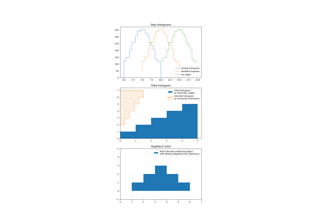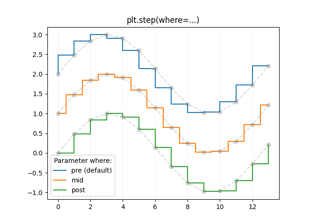# matplotlib.pyplot.step#

matplotlib.pyplot.step(x, y, *args, where='pre', data=None, **kwargs)[source]#

Make a step plot.

Call signatures:

```step(x, y, [fmt], *, data=None, where='pre', **kwargs)
step(x, y, [fmt], x2, y2, [fmt2], ..., *, where='pre', **kwargs)
```

This is just a thin wrapper around `plot` which changes some formatting options. Most of the concepts and parameters of plot can be used here as well.

Note

This method uses a standard plot with a step drawstyle: The x values are the reference positions and steps extend left/right/both directions depending on where.

For the common case where you know the values and edges of the steps, use `stairs` instead.

Parameters:
xarray-like

1D sequence of x positions. It is assumed, but not checked, that it is uniformly increasing.

yarray-like

1D sequence of y levels.

fmtstr, optional

A format string, e.g. 'g' for a green line. See `plot` for a more detailed description.

Note: While full format strings are accepted, it is recommended to only specify the color. Line styles are currently ignored (use the keyword argument linestyle instead). Markers are accepted and plotted on the given positions, however, this is a rarely needed feature for step plots.

where{'pre', 'post', 'mid'}, default: 'pre'

Define where the steps should be placed:

• 'pre': The y value is continued constantly to the left from every x position, i.e. the interval `(x[i-1], x[i]]` has the value `y[i]`.

• 'post': The y value is continued constantly to the right from every x position, i.e. the interval `[x[i], x[i+1])` has the value `y[i]`.

• 'mid': Steps occur half-way between the x positions.

dataindexable object, optional

An object with labelled data. If given, provide the label names to plot in x and y.

**kwargs

Additional parameters are the same as those for `plot`.

Returns:
list of `Line2D`

Objects representing the plotted data.

## Examples using `matplotlib.pyplot.step`#Stairs Demo

Stairs DemoStep Demo

Step Demo